# Matrix delivery system

In a matrix or monolithic delivery system the drug is either molecular dissolved or dispersed inside a matrix. Compared to a reservoir system, a matrix system is not enveloped within a rate limiting membrane. As such the release rate of the drug from the matrix system is normally not constant and decreases in time.  In literature various types of matrix systems are described. In principle they can be categorized in:

• Diffusion based designs (homogenous matrix, porous matrix)
• Dissolution based designs
• Erosion based designs (bulk erosion or surface erosion)
• Combination of diffusion/dissolution based designs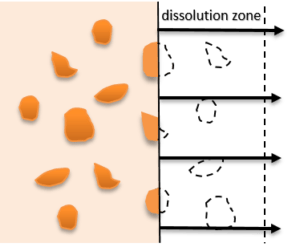diffusion based matrix system dissolution/surface erosion based matrix system

## Diffusion based matrix system

In a diffusion based design the release of a drug is mainly influenced by the diffusion properties of the drug in the matrix. In contrast to a dissolution based matrix system, the matrix itself is inert and does not dissolve nor degrade in the dissolution medium. This means that the dimensions of the matrix do not change during release of the drug.

Fick’s laws of diffusion describe diffusion process of molecules in a matrix and were derived by Adolf Fick in 1855. For steady state conditions the diffusion process is described by Fick first law of diffusion which states that the drug in solution passing a unit area is proportional to the concentration difference over this unit area:Where:
J=flux (kgm-2s-1)
dC/dx=concentration difference (kgm-4)
D=Diffusion constant (m2s-1)

For non-steady state diffusion Fick’s second law describes how diffusion causes the concentration to change with time: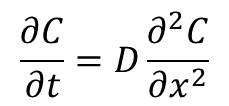In literature two types of matrix systems are generally mentioned in literature i.e. homogenous and porous matrix systems.

Homogeneous matrix

A homogeneous matrix system the drug is either dissolved or dispersed in a homogeneous matrix, mostly a polymer. Once the matrix system is immersed in a dissolution medium the drug, present at the surface of the matrix, will dissolve in the dissolution medium. This will result in a concentration difference and drug will diffuse from the inner layers to the outer layers of the matrix. As a result a depletion zone will be formed at the boundary of the matrix which increases in time. Because the drug has to diffuse over a longer distance the release rate will also decrease in time.

The release of a drug from a planar matrix system under sink conditions was described by Higuchi in 1961:Where: Q = the amount of drug released (M) per surface area (A)
D = the diffusion coefficient of the drug
C0 = is the initial total drug concentration in the matrix
Cs = the solubility concentration of the drug in the matrix
t  =  time

 This equation can be simplified to:It shows that the release rate is inverse proportional to the square root of time. If the release rate is plotted versus the square root of time a straight line is obtained.

Porous matrix system

A porous matrix system is a variation of a homogenous matrix system. In this case the drug is dissolved in a porous polymer. Once the matrix system is immersed in a dissolution medium the pores will be filled with dissolution medium and the drug will dissolve into the filled pores of the matrix. Besides the solubility and diffusion coefficient of the drug in the release medium also the shape and volume of the pores in the matrix are of importance. Under sink conditions the release rate can be described by:Where:
τ = tortuosity
ϵ = porosity of the matrix

Here tortuosity is defined as the dimensions and branching of the pores in the matrix.

Power law equation

It should be noted that the Higuchi equations are only valid for matrices that are inert to the dissolution medium. If the matrix swells, dissolves or if the diffusivity changes in times the Higuchi equations are not accurate anymore. In order to predict the release from these systems Korsemeyer and Peppas (1983) derived a simple relationship also known as the The Power Law: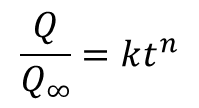Where:
Q=the amount of drug released at time t
Q=the amount of drug released at infinite time
n=diffusional exponent

The next figure shows two cylindrical matrix delivery systems with their corresponding release plots. The release was calculated using finite element software for an arbitrary drug which was homogeneous dispersed in a matrix based implant (Ø2mmx20mm) at a concentration of 50 wt% (green line). In order to tune the amount of drug needed to the desired duration of the matrix delivery system, it is possible to only load a small layer at the boundary of the implant. This was demonstrated for a thin outer layer (blue line). In this way no more drug is used than needed.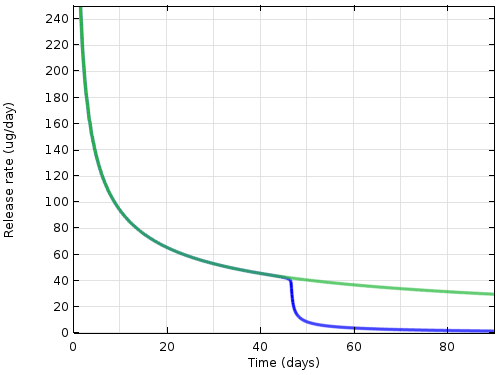## Dissolution based matrix system

In contrast to a diffusion based design the release rate of drug from a dissolution based design is mainly determined by the slow dissolution of the matrix in a dissolution medium. Once the matrix system is immersed in dissolution medium the matrix will slowly dissolve thereby releasing the drug. This means that the dimensions of a dissolution based matrix design will alter in time. In case spherical or cylindrical based matrix designs the drug release rate will also decrease in time.

Noyes and Whitney equation

The basic equation for dissolution of a matrix was described by Noyes and Whitney.  They state that the dissolution rate at which a planar matrix dissolves is proportional to the difference in saturation solubility and the concentration in the dissolution medium.This basic equation was later modified by Nernst and Brunner:Where: dM/dt = dissolution rate
D = diffusion coefficient
A = surface area
h= thickness stagnant layer
Cs = saturation solubility
C = concentration in the dissolution medium

Hixson & Crowell equation

Because the release of drug is proportional to the surface area of the matrix system the geometry of the matrix system is of influence.  For a spherical dissolution based matrix system the release of drug can be described by the Hixson & Crowell equation. This equation is also known as the cube root law and describes the release from systems where there is a change in surface area and diameter of particles.
When the cube root of the amount of drug released is plotted versus time a straight line is obtained.Where:
W0=the initial amount of drug
Wt=the amount of drug at time t
Ks=constant (this constant contains information as density of the matrix and solubility)

## Erosion based matrix system

In an erosion based matrix system the drug is mainly released when the matrix in which the drug is dissolved or dispersed erodes either by bulk erosion or by surface erosion.

The release mechanisms based on polymer erosion are complex and it has been difﬁcult to describe the release process in simple equations. As in case of diffusion controlled matrix systems, the release of a drug from erosion based matrix system also depends on the geometry of the delivery system.

Since surface erosion is easier to control than bulk erosion, surface erosion is preferred in drug delivery. The following mathematical expression has been described by Hopfenberg to predict the drug release from simple surface eroding geometries. The cumulative fraction of drug released at time t was described as: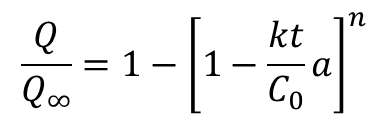Where
Q=the amount of drug released at time t
Q=the amount of drug released at infinite time
a= system’s half thickness (i.e. the radius for a sphere or cylinder),
n= exponent that varies with geometry n = 1, 2, 3 (i.e. slab, cylindrical, spherical geometry)

## References

Fick, A., 1855. Uber diffusion, Annalen der Physik 94, 59–86.
Higuchi,T.,1961.Rate of release of medicaments from ointment bases containing drugs in suspensions, Pharm. Sci. 50, 874–875.
Higuchi, T., 1963. Mechanisms of sustained action mediation , Theoretical analysis of rate of release of solid drugs dispersed in solid matrices,  J.Pharm., Sci. 52, 1145–1149
Hixson  A.W.,  Crowell  J.H.:  Ind.  Eng.  Chem. 23, 923 (1931).
Noyes A.A., Whittney W.R.: J. Am. Chem. Soc.19, 930 (1897)
Peppas, N.A., 1985. Analysis of Fickian and non-Fickian drug release from polymers. Pharm. Acta Helv. 60, 110–111.
Korsmeyer, R.W., Peppas, N.A., 1983b. Modeling drug release from swellable systems. Proc. Symp. Control. Release Bioact. Mater. 10, 141–144.
Costa P.: Int. J. Pharm., 220, 77 (2001)
Hopfenberg   H.B.:   in   Controlled   Release Polymeric Formulations, Paul D.R, Haris F.W. Eds.,   (ACS Symposium   Series   No.   33), American Chemical Society, Washington 1976.